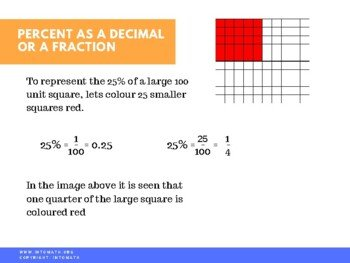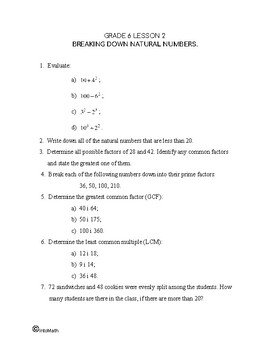CA$2.00 Percent is a concept that is widely used in everyday life in a variety of situations. “30% Discount”, “Yes, definitely, 100%” – are just some of the expressions that we encounter frequently. It is important to understand what the above means and how it can be useful. A percent is a number out of a 100. The term “percent” is derived from the Latin per centum, meaning “by the hundred”. In Ancient Rome, before the decimal system was invented, computations were often made in fractions which were multiples of 1/100 (or 0.01 if recorded as a decimal). Thus, computation with these fractions was the same as computing percentages.Grade 6 Workbook (16 pages with answers) CA$4.00

100% represents a whole and can be split into parts: 25%, 40%, 78%, etc.

Percent was invented for convenience in order to easier represent fractions or decimals. Therefore, it can be converted into a fraction or a decimal.

For example, 1% = 0.01, because to convert a percent into a decimal, we need to divide the value by 100%.

We use percent to solve real world problems. For example, when we  calculate the grade, we do so out of 100. Or when we survey people and show their preferences. We usually use a pie graph, split into parts, where one complete circle represents 100. We also use the concept when we discuss the improvement or when we measure something. In this lesson you will see how to do that.